# Grid customization in ggplot2

By default, ggplot2 creates a major and a minor white grid as shown in the following figure.

``````library(ggplot2)
ggplot(data = mtcars, aes(x = hp, y = mpg))+
geom_point()``````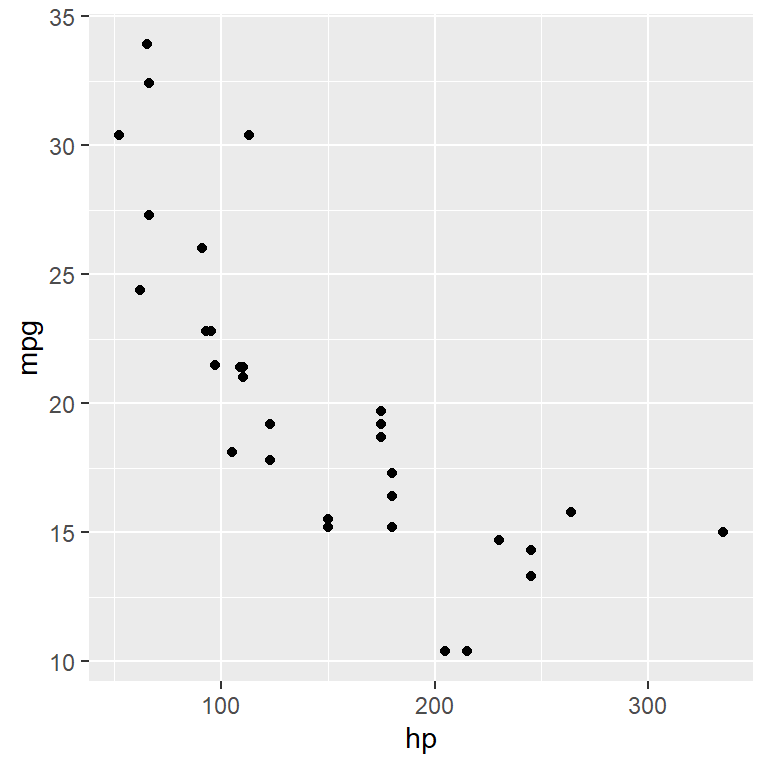## Grid customization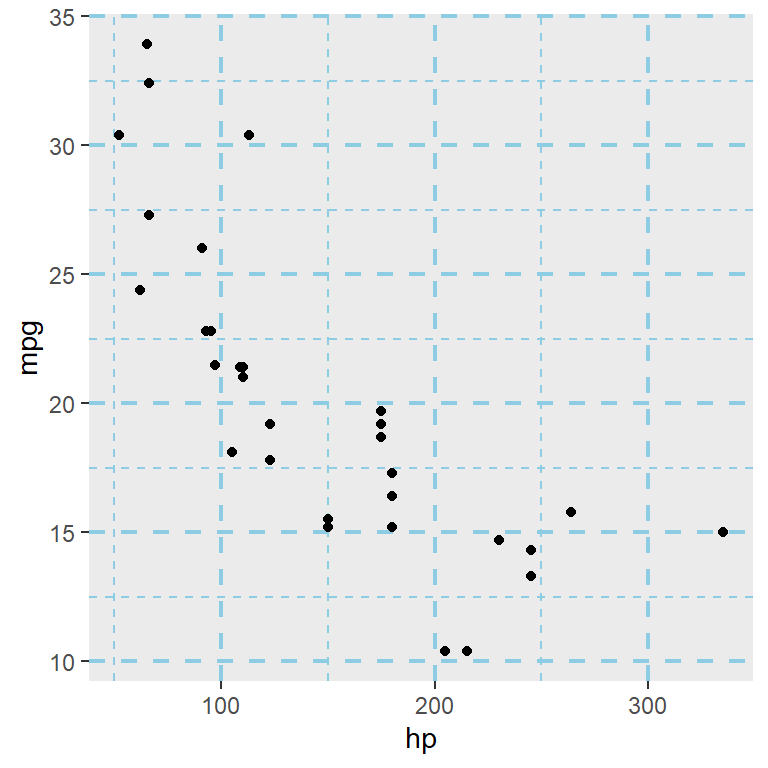The grid aesthetics can be set with the `panel.grid` component of the `theme` function. Customize the color, line width and line type with the arguments of the `element_line` function.

``````library(ggplot2)

ggplot(data = mtcars, aes(x = hp, y = mpg)) +
geom_point() +
theme(panel.grid = element_line(color = "#8ccde3",
size = 0.75,
linetype = 2))``````

You can also customize the major grid and the minor grid individually, as shown in the following sections.

## Major grid

The `panel.grid.major` allows you to customize the major grid of the panel.

``````library(ggplot2)

ggplot(data = mtcars, aes(x = hp, y = mpg)) +
geom_point() +
theme(panel.grid.major = element_line(color = "red",
size = 0.5,
linetype = 2))``````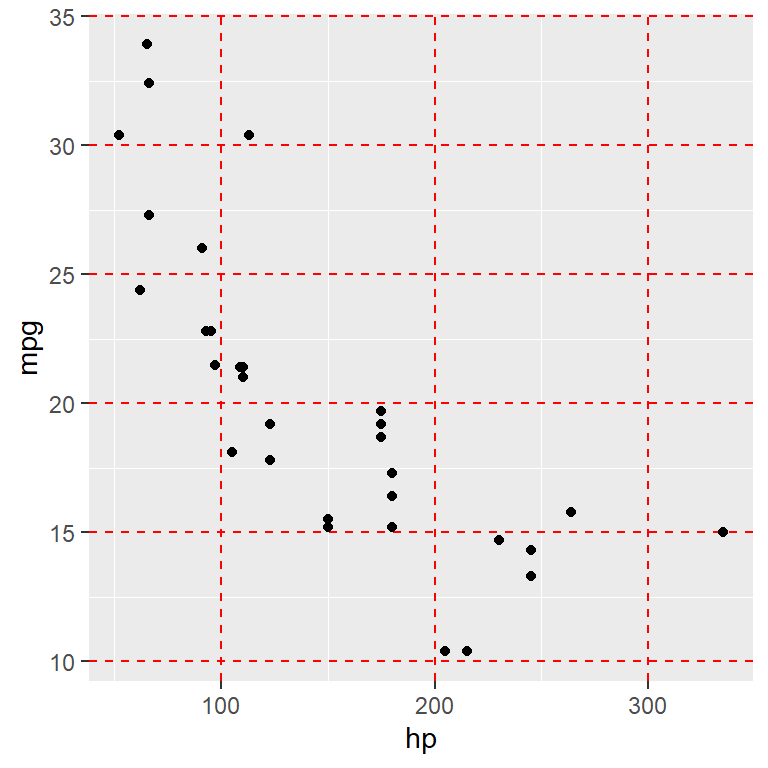Appending `.x` or `.y` to the previous component will allow customizing the vertical and horizontal lines of the major grid.

Vertical lines of the major grid

``````library(ggplot2)

ggplot(data = mtcars, aes(x = hp, y = mpg)) +
geom_point() +
theme(panel.grid.major.x = element_line(color = "red",
size = 0.5,
linetype = 2))``````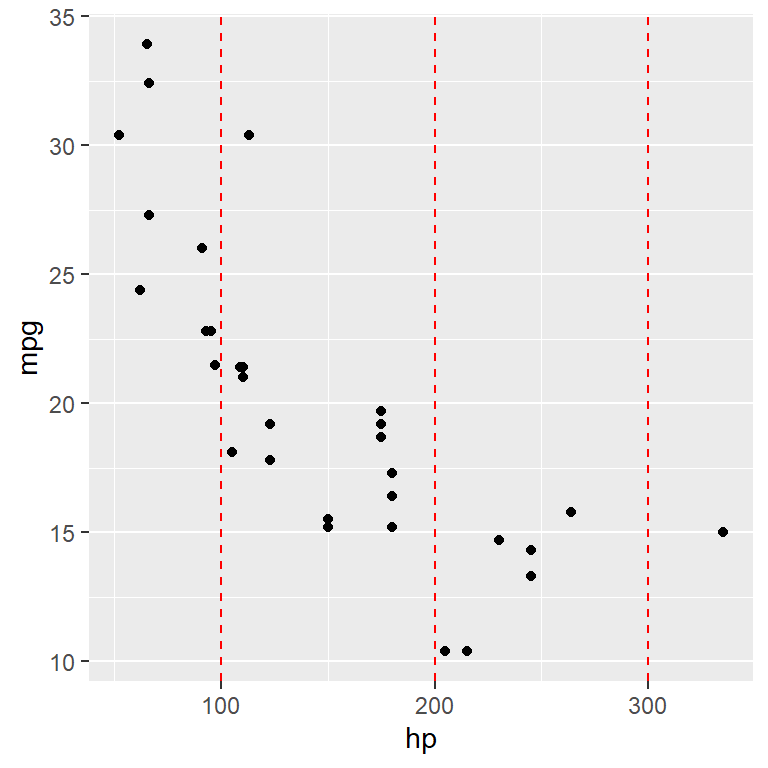Horizontal lines of the major grid

``````library(ggplot2)

ggplot(data = mtcars, aes(x = hp, y = mpg)) +
geom_point() +
theme(panel.grid.major.y = element_line(color = "red",
size = 0.5,
linetype = 2))``````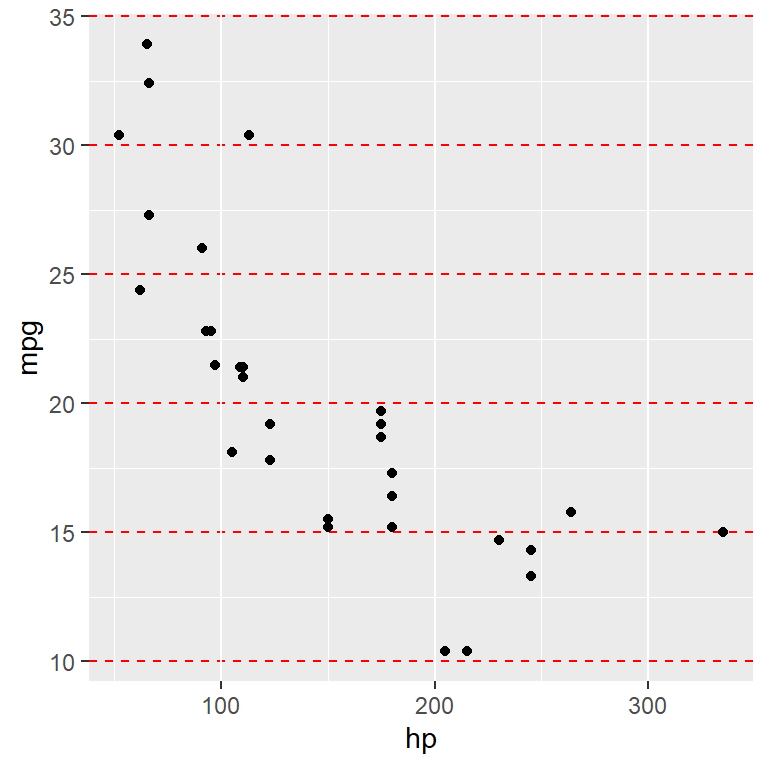## Minor grid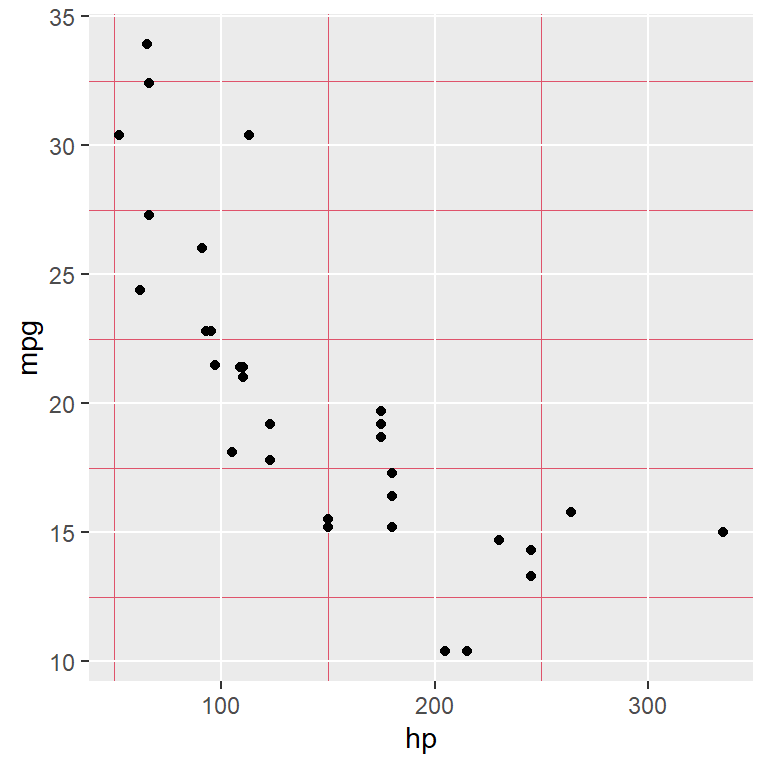The `panel.grid.minor` allows you to customize the minor grid of the panel.

``````library(ggplot2)

ggplot(data = mtcars, aes(x = hp, y = mpg)) +
geom_point() +
theme(panel.grid.minor = element_line(color = 2,
size = 0.25,
linetype = 1))``````

As in the previous section, you can append `.x` or `.y` to the theme element.Vertical lines of the minor grid

``````library(ggplot2)

ggplot(data = mtcars, aes(x = hp, y = mpg)) +
geom_point() +
theme(panel.grid.minor.x = element_line(color = 2,
size = 0.25,
linetype = 1))``````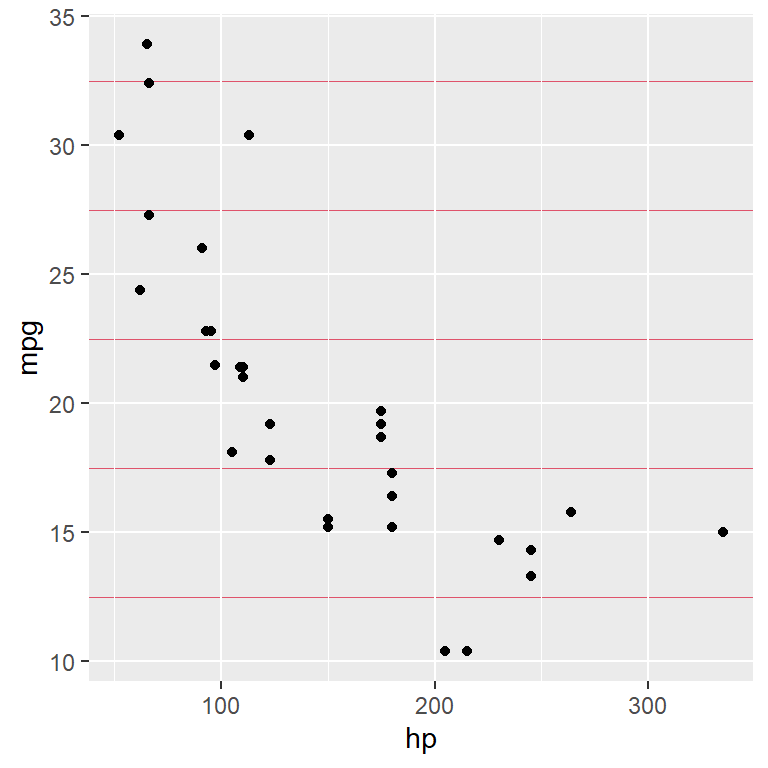Horizontal lines of the minor grid

``````library(ggplot2)

ggplot(data = mtcars, aes(x = hp, y = mpg)) +
geom_point() +
theme(panel.grid.minor.y = element_line(color = 2,
size = 0.25,
linetype = 1))``````

## Custom grid breaks

The number of grid breaks can be customized in ggplot for each axis with the `breaks` argument of the `scale_(x|y)_continuous` or `scale_(x|y)_discrete` functions, depending on if the variable of the `(x|y)`-axis is continuous or discrete.

In this example you can customize the breaks of the Y-axis with the `scale_y_continuous` function as follows.

``````library(ggplot2)

ggplot(data = mtcars, aes(x = hp, y = mpg)) +
geom_point() +
scale_y_continuous(breaks = seq(10, 35, by = 1))``````As the X-axis is also continuous you can make use of the `scale_x_continuous` to customize the grid breaks of the X-axis.

``````library(ggplot2)

ggplot(data = mtcars, aes(x = hp, y = mpg)) +
geom_point() +
scale_x_continuous(breaks = seq(50, 350, by = 25))``````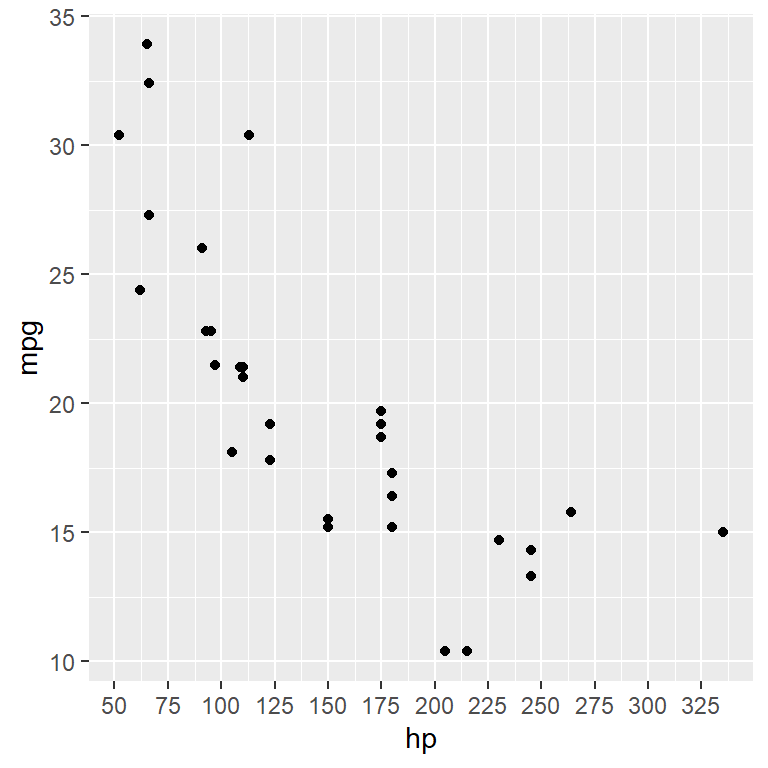Set minor breaks with `minor_breaks` argument.

``````library(ggplot2)

ggplot(data = mtcars, aes(x = hp, y = mpg)) +
geom_point() +
scale_x_continuous(breaks = seq(50, 350, by = 25),
minor_breaks = seq(50, 350, 10))``````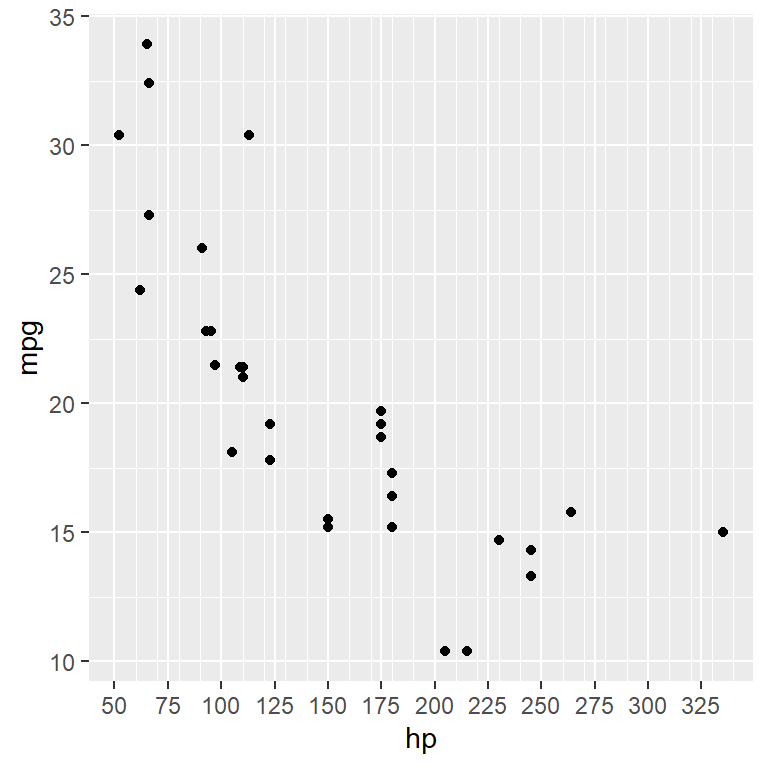Set the number of major breaks with `n.breaks` argument.

``````library(ggplot2)

ggplot(data = mtcars, aes(x = hp, y = mpg)) +
geom_point() +
scale_x_continuous(n.breaks = 20)``````

The algorithm behind the generation of major breaks may choose a different number than the specified to ensure nice break labels.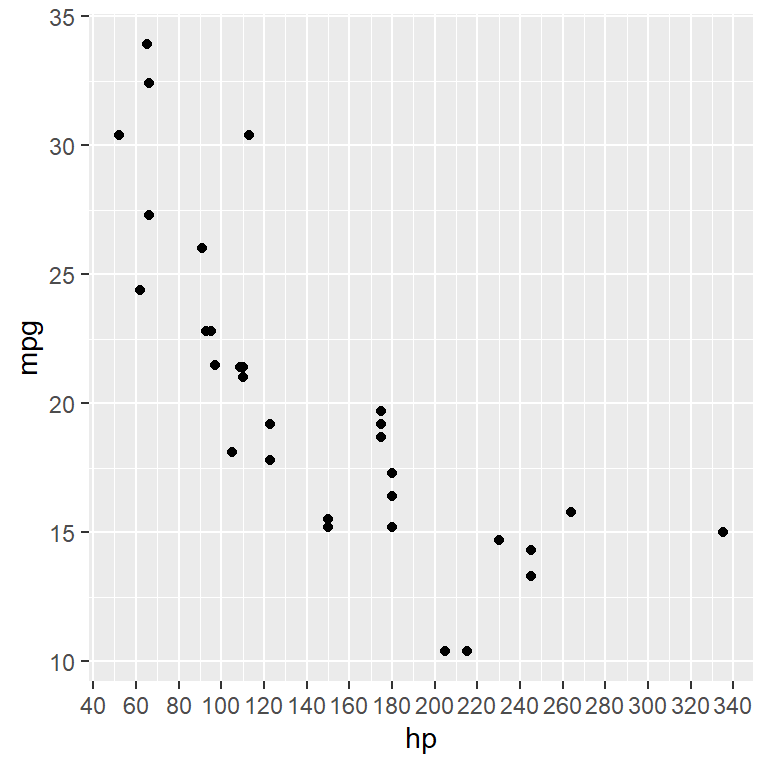## Remove grids

The same way you customized each grid (`panel.grid`, `panel.major`, `panel.major.x`, `panel.major.y`, `panel.minor`, `panel.minor.x`, `panel.minor.y`) you can remove them but setting `element_blank` instead of `element_line`.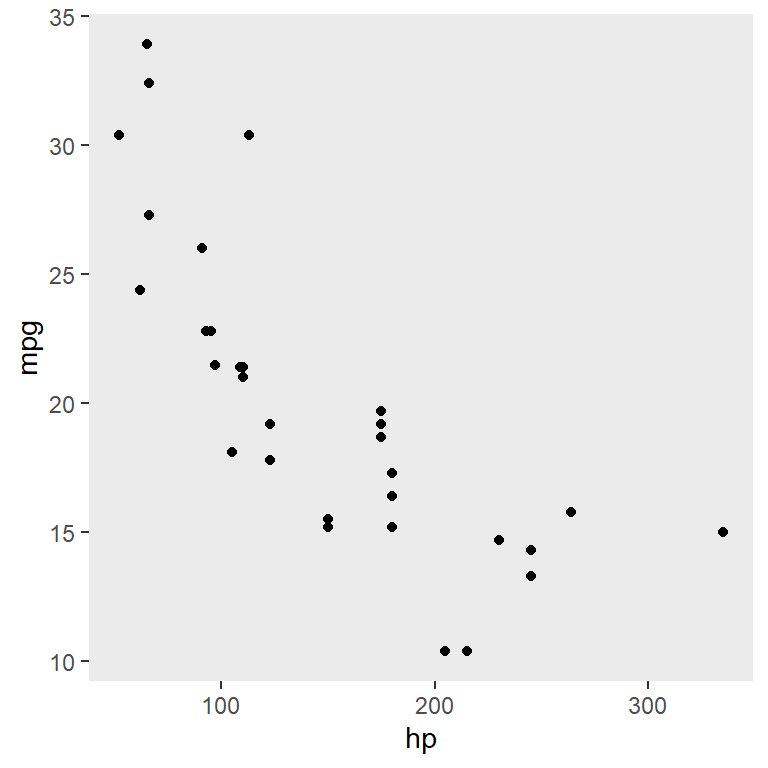Remove all grids

``````library(ggplot2)

ggplot(data = mtcars, aes(x = hp, y = mpg)) +
geom_point() +
theme(panel.grid = element_blank())``````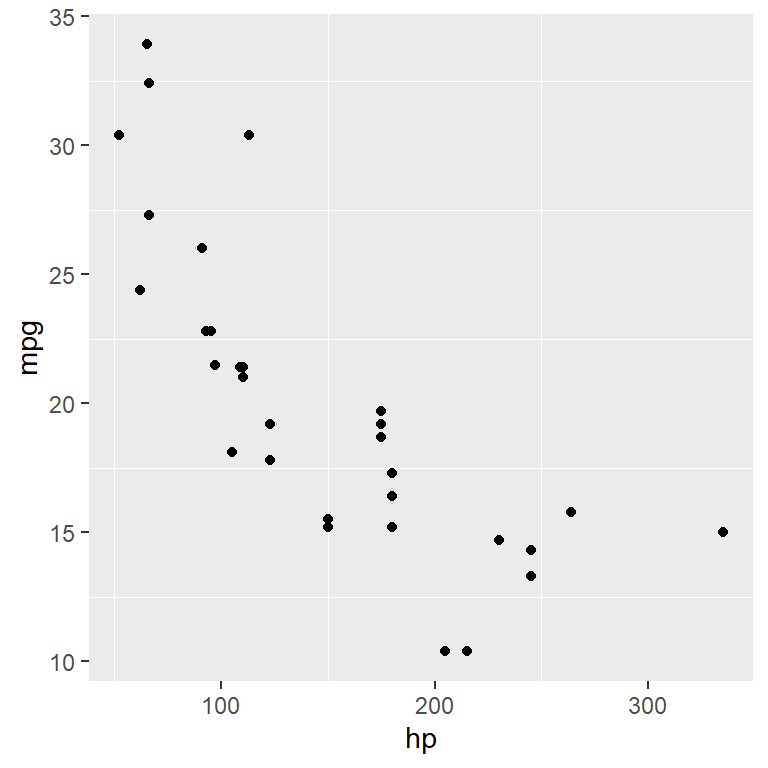Remove the major grid

``````library(ggplot2)

ggplot(data = mtcars, aes(x = hp, y = mpg)) +
geom_point() +
theme(panel.grid.major = element_blank())``````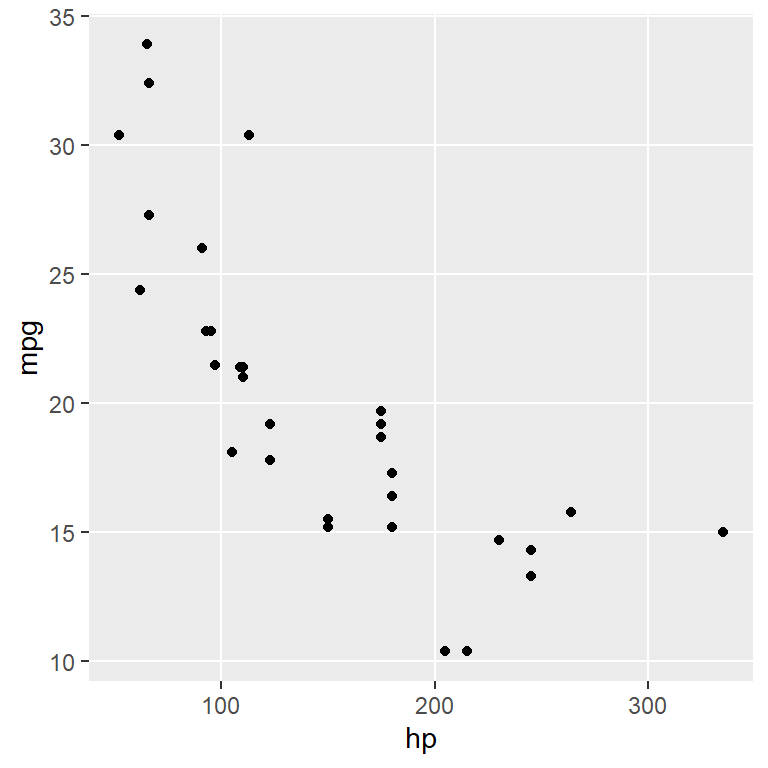Remove the minor grid

``````library(ggplot2)

ggplot(data = mtcars, aes(x = hp, y = mpg)) +
geom_point() +
theme(panel.grid.minor = element_blank())``````